Share
Explore

Approaches
> POC from Covidae.ai
> Our own model (Markov v/s Shapley)

Markov
Get user data of timestamp, cookie ID and channel of interaction (do we collect this data?)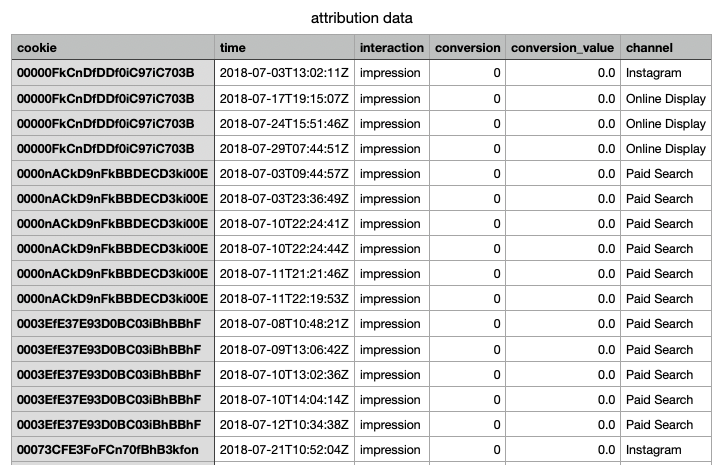Format to get all user journeys in order of timestamp per unique cookie id to get user journey like [start, Facebook, Instagram, google non-brand, null]
Null here is if no conversion event took place or can be other values eg; at the lead level in our case as there would be enough data of that level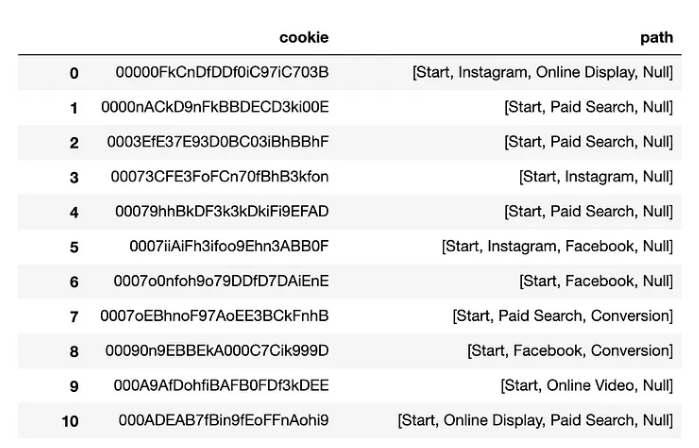Get transition probabilities using Markov chains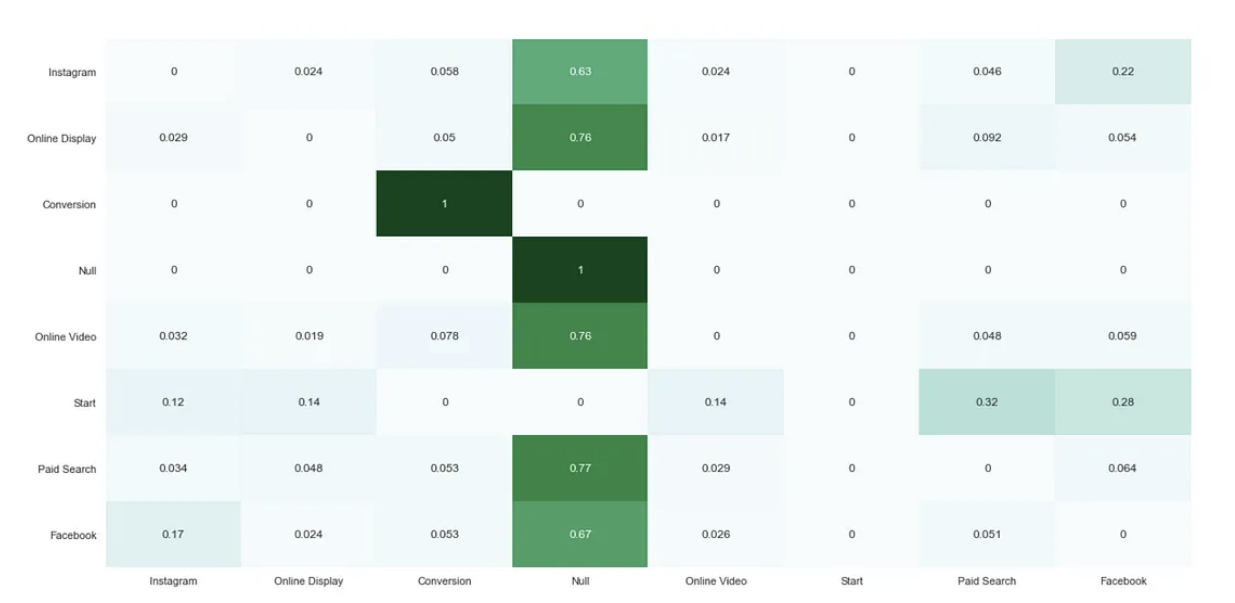Now basis whatever the new combination of user touch points in a matrix, we can multiply that with this matrix to get the probability of conversion for that user journey
We can now iteratively go through each of our channels and assess the impact it would have on overall conversion if we were to remove a channel from our state-space.
The resulting removal effects dictionary can then be used to calculate the Markov Chain attributions for each of our marketing channels: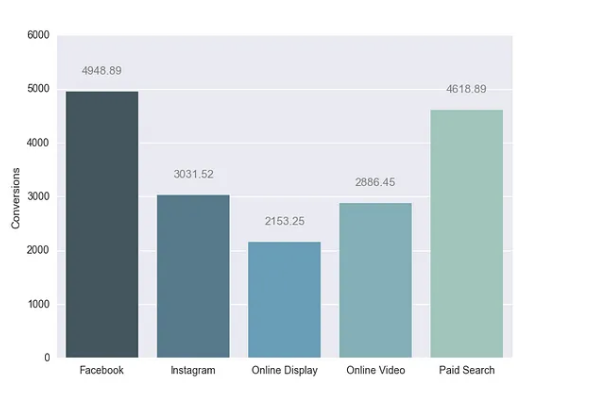The Markov attribution can be done with the following dimensions; channel, campaign

Shapley
Comes from game theory for distribution of team player’s contribution in goals
The characteristic function
A game is defined by a set of players N and a characteristic function v. Every subset of players is called a coalition S, and the characteristic function v(S) assigns a value to each coalition to signify its worth. A coalition’s worth represents the payoff that it can generate when its players work together.
Options for defining the characteristic function for marketing include:
Total revenue generated by each coalition
Total number of sales conversions generated by each coalition
Conversion ratio of each coalition (conversions / opportunities)
Conditional probability of conversion — likelihood of converting given a set of channels
With conversion Ratios:
Find conversion ratios of all channel combination from actual user journeys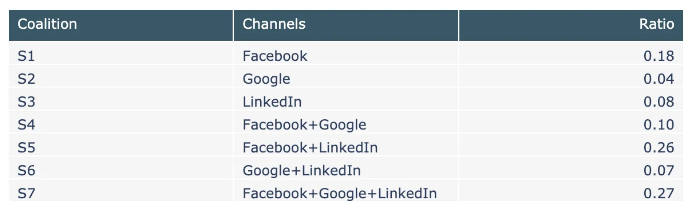Use the characteristic function which is the sum of all conversion ratios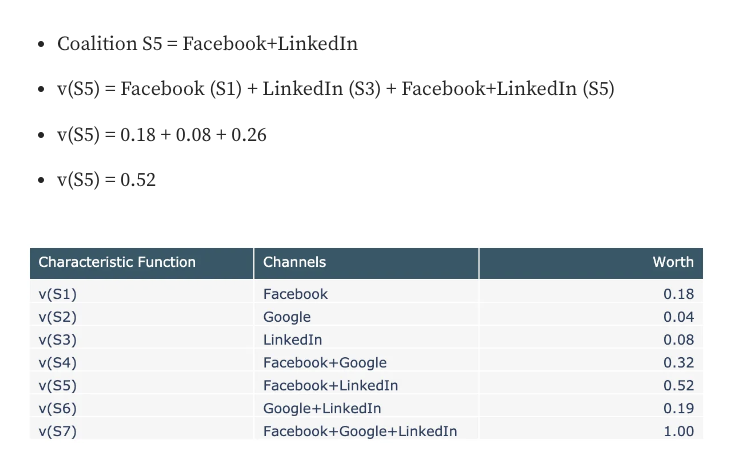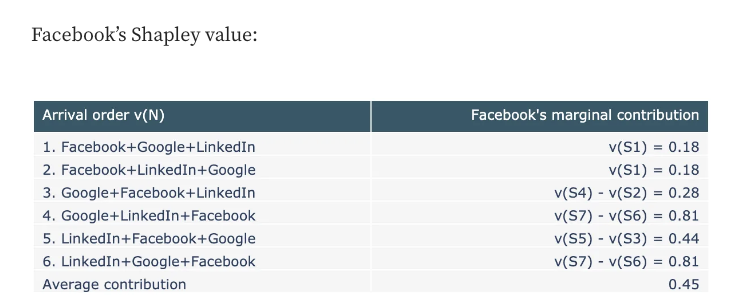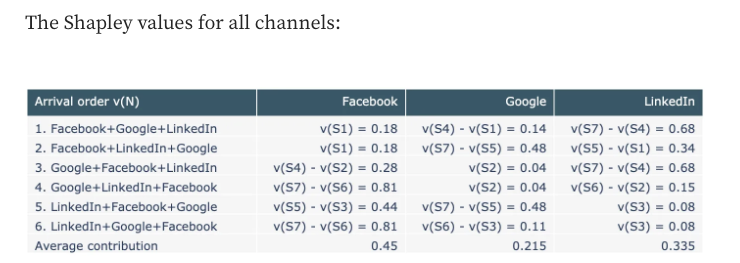Conversion Ratios of each channel: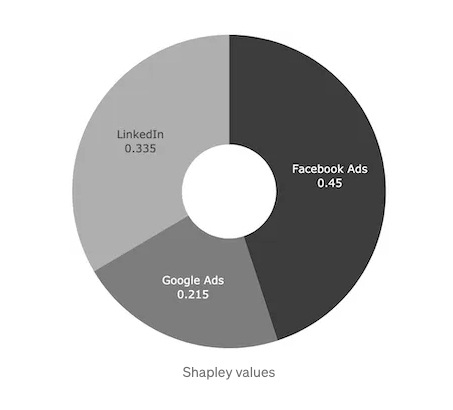Steps
Get data on time stamp, channel, cookie id and conversion (0/1)
Make user journeys like explained before with 0 and 1 at the end
Now find conversion probabilities of these journeys converting
For any new set of user journey we can add/substract conversion ratios to get the value of any new interaction mix to calculate it’s worth
Now that we know the worth of each coalition, the Shapley values can be calculated by taking the average of each channel’s marginal contribution to the game, accounting for all possible orderings. Specifically, the Shapley value gives us a way to distribute the worth of the grand coalition (total payoff) between the three channels.

Caveat: Since we would need significant data for these unique user journeys, we would need to do this at a lead level
We can do it at a campaign and channel level data for now and try both approaches